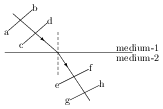# Huygen's Principle

## Problems from IIT JEE

PARAGRAPH (IIT JEE 2007):The figure shows a surface XY separating two transparent media, medium-1 and medium-2. The lines ab and cd represent wavefronts of a light wave travelling in medium-1 and incident on XY. The lines ef and gh represents wavefronts of the light wave in medium-2 after refraction.

Question 1: Light travels as a,

1. parallel beam in each medium.
2. convergent beam in each medium.
3. divergent beam in each medium.
4. divergent beam in one medium and convergent beam in other medium.

Solution: The wavefronts in medium 1 are parallel to each other. Hence incident beam consists of parallel light rays. Same is true for refracted beam in the medium 2. Thus, A is correct.

Question 2: The phases of the light wave at c, d, e and f are $\phi_c$, $\phi_d$, $\phi_e$ and $\phi_f$ respectively. It is given that $\phi_c\neq \phi_f$.}

1. $\phi_c$ cannot be equal to $\phi_d$.
2. $\phi_d$ can be equal to $\phi_e$.
3. $(\phi_d-\phi_f)$ is equal to $(\phi_c-\phi_e)$.
4. $(\phi_d-\phi_c)$ is not equal to $(\phi_f-\phi_e)$.

Solution: By definition, the phase at all points on a wavefront is equal. Thus, $\phi_c=\phi_d$ and $\phi_e=\phi_f$. Hence, $(\phi_d-\phi_f)=(\phi_c-\phi_e)$. Also, since $\phi_c\neq\phi_f$, we get $(\phi_c=\phi_d)\neq (\phi_e=\phi_f)$. Thus C is correct option.

Question 3: Speed of light is,

1. the same in medium-1 and medium-2.
2. larger in medium-1 than in medium-2.
3. larger in medium-2 than in medium-1.
4. different at b and d.

Solution:The ray of light travels normal to the wavefront. The ray bends towards the normal while going from medium 1 to medium 2. Hence, by Snell's law, medium 2 is denser than medium 1 i.e., $\mu_2 > \mu_1$. Thus, $v_2 = c/\mu_2 < c/\mu_1 = v_1$ i.e., speed of light is larger in medium 1. Thus B is correct choice.